この節の作者: Rebecca Vederhus, Sebastian Jentschke

# From SPSS to jamovi: Non-parametric analyses¶

This comparison shows how a Wilcoxon signed-rank test is conducted in SPSS and jamovi. The SPSS test follows the description in chapter 7.5.2 in Field (2017), especially figure 7.9 and output 7.3 - 7.4. It uses the data set Drug.sav which can be downloaded from the web page accompanying the book.
SPSS jamovi
In SPSS, first split the file using `Data``Split File`. In jamovi, the file can be split using: `Variables``Filters`. In the window that appears, write `= Drug == "Ecstasy"` and then save the output. Open the original file again and repeat these steps but write the following command instead: `= Drug == "Alcohol"`. Now you will have two separate files, one for each drug.In the `Split File` window, click `Organize output by groups`. Then, move the `Drug` variable to the box called `Groups Based on`. Lastly, click `Sort the file by grouping variables`.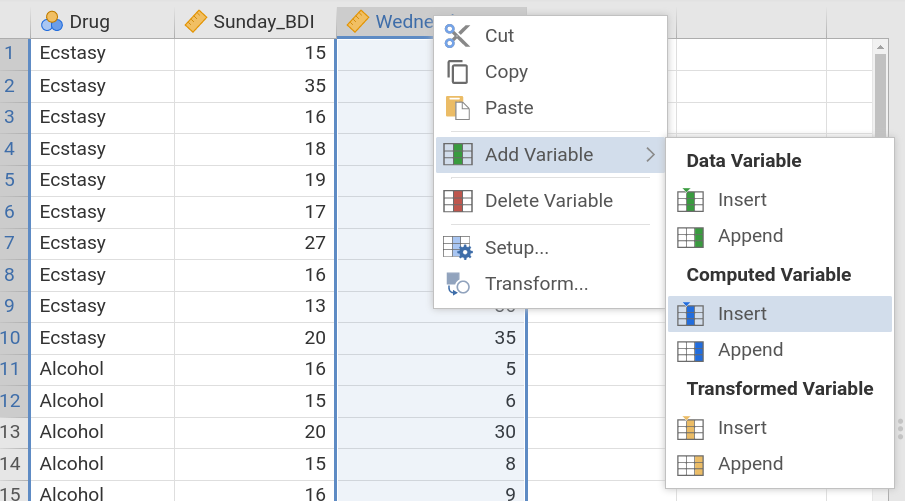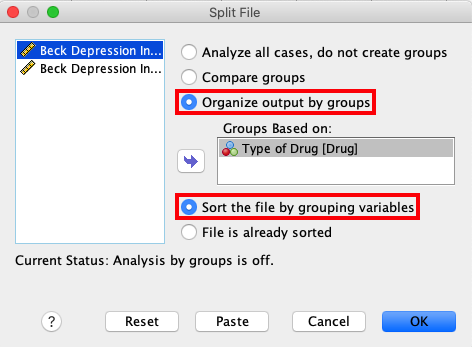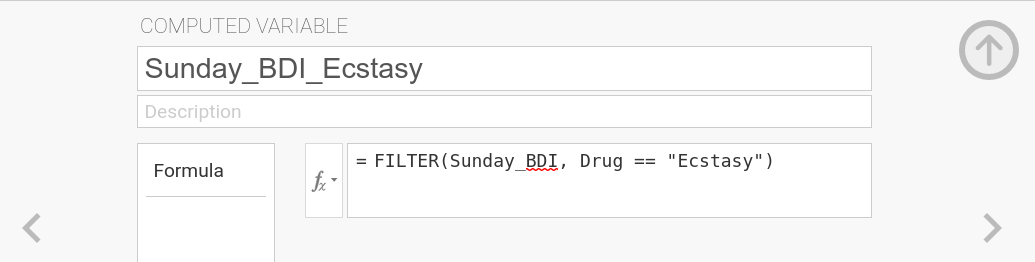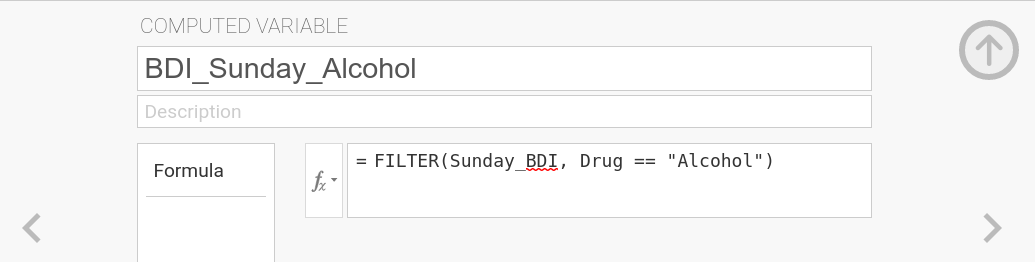Then, create a Mann-Whitney test using: `Analyze``Nonparametric Tests``Related Samples`. Within each file, use the following steps to create a Wilcoxon signed-rank test: `Analyses``T-tests``Paired Samples T-Test`.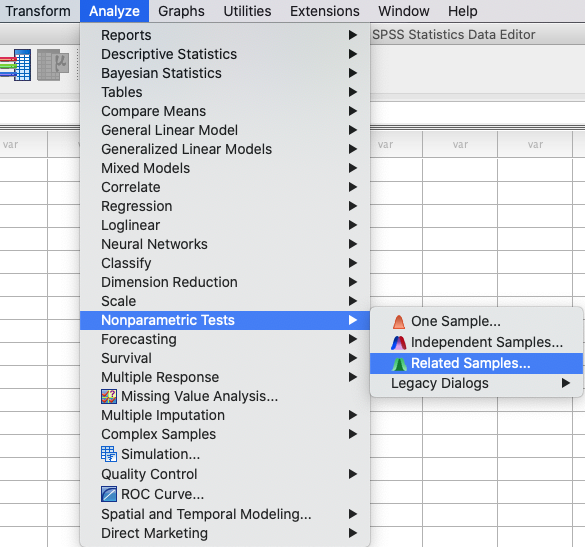In the `Nonparametric Tests` window, open `Fields` and click ```Use custom field assignments```. Move the `Sunday` and `Wednesday` variables to the `Test Fields` box. In jamovi, mark `Sunday_BDI` and `Wednesday_BDI` and move them to the `Paired Variables` box. Then, tick the box for `Wilcoxon rank`. Repeat this procedure for both output files.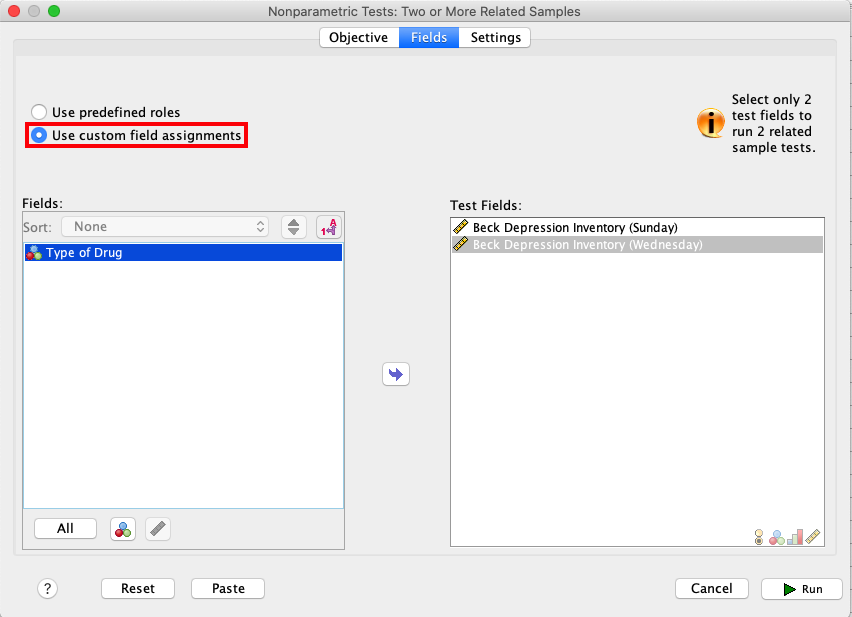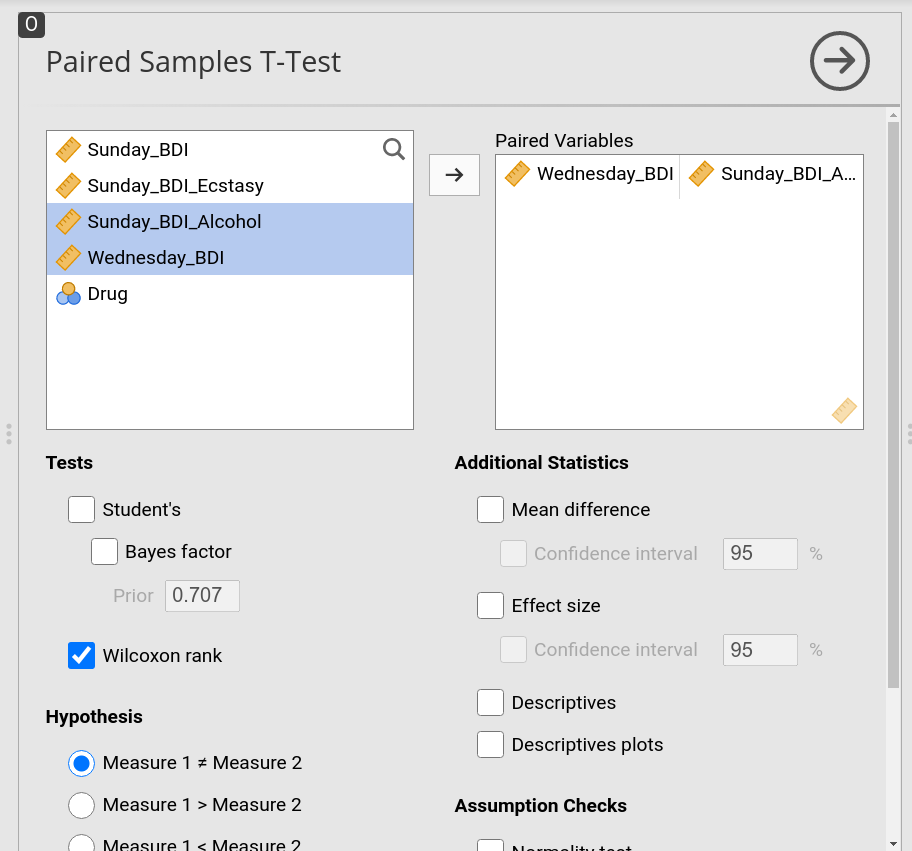Lastly, open `Settings` and click `Customize tests`. Tick the box for `Wilcoxon matched-pair signed-rank` as shown in the picture below.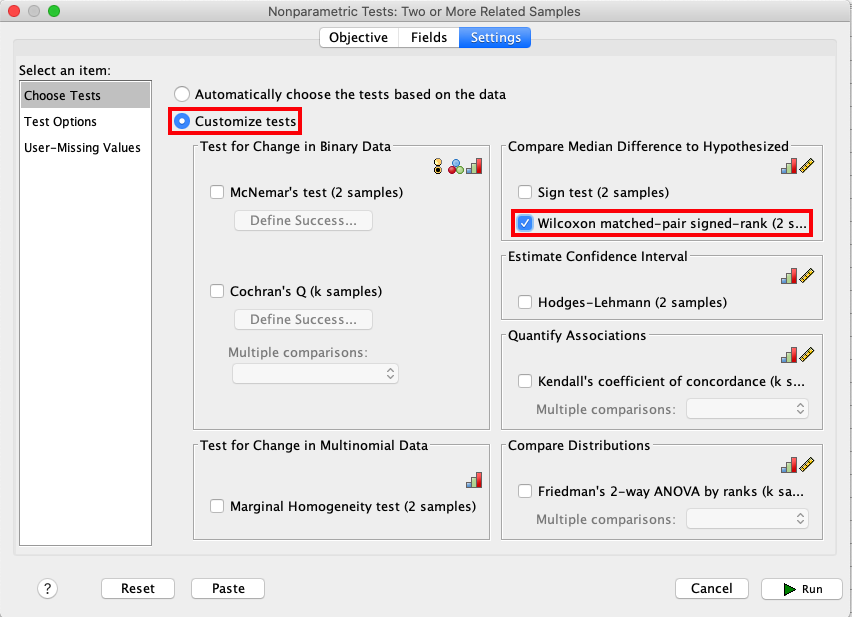Comparisons of the output show that the results in SPSS and jamovi are slightly different.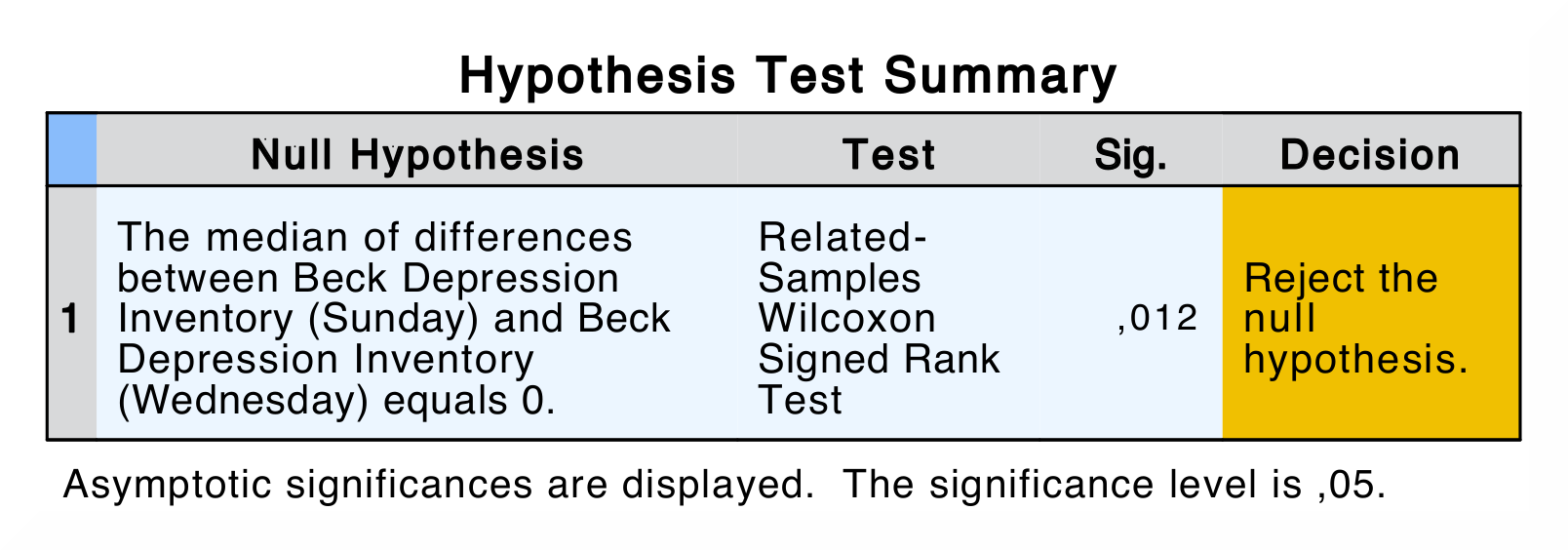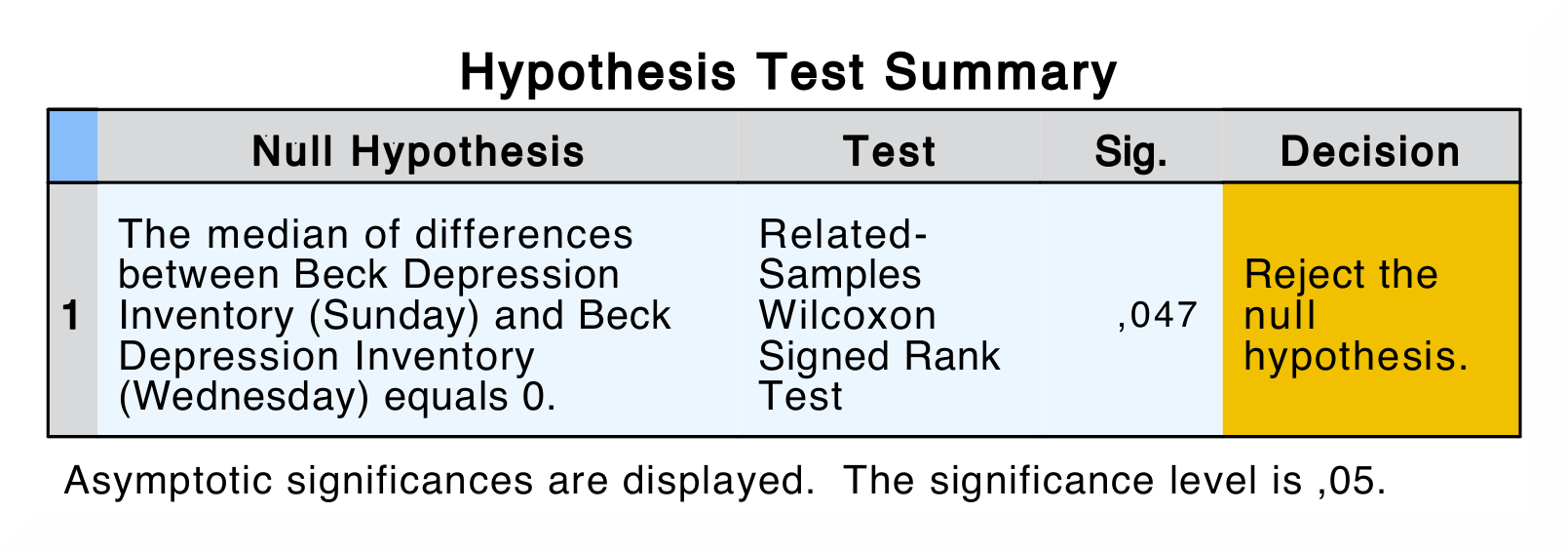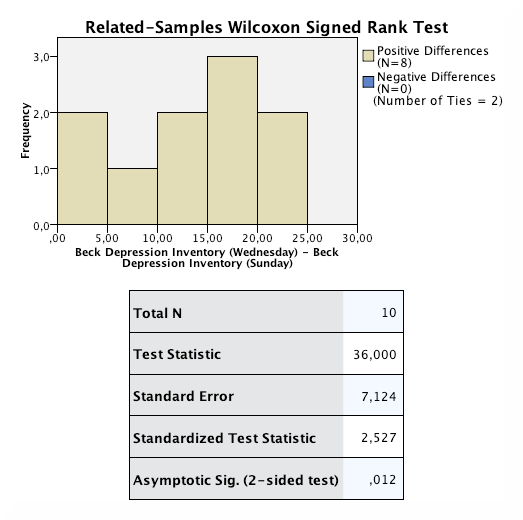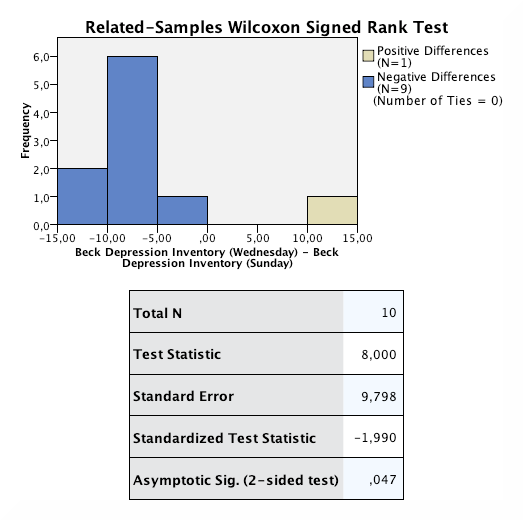In SPSS, the first output table is the model summary, which only shows the p-value for the test. If you double-click this table, you get the details of the analysis. There are two model summaries, on for each drug condition. The Wilcoxon Signed Rank test show the following values: `Total N`, ```Test Statistic```, `Standard Error`, `Standardized Test Statistic` and `Asymptotic Sig. (2-sided test)`. In jamovi, there are two output files, with a table each. In contrast to SPSS, the `Paired Samples T-Test` table only displays the test statistic and its significance value.
When comparing the output, none of the numerical values are the same. The reason for the difference in p-values is that jamovi applies continuity correction (where a discrete distribution – ranks – is approximated via a continuous one – the standard normal distribution) whereas SPSS doesn't.
If you wish to replicate those analyses using syntax, you can use the commands below (in jamovi, just copy to code below to Rj). Alternatively, you can download the SPSS output files and the jamovi files with the analyses from below the syntax.
```NPTESTS
/RELATED TEST(Sunday_BDI Wednesday_BDI) WILCOXON
/MISSING SCOPE=ANALYSIS USERMISSING=EXCLUDE
/CRITERIA ALPHA=0.05 CILEVEL=95.
```
```jmv::ttestPS(
data = data,
pairs = list(
list(i1 = "Sunday_BDI", i2 = "Wednesday_BDI")),
students = FALSE,
wilcoxon = TRUE)
```
SPSS output file containing the analyses jamovi file with the analyses for Alcohol jamovi file with the analyses for Ecstasy
References
Field, A. (2017). Discovering statistics using IBM SPSS statistics (5th ed.). SAGE Publications. https://edge.sagepub.com/field5e